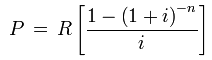# Annuity

Financial analysis Print Email

In finance theory, the term annuity is used to refer to a terminating stream of fixed payments over a particular time period. Most commonly, this usage is reflected in financial discussions, generally in connection with the valuation of the payments’ stream, also considering the time value of money concepts like interest rates and future value. The annuities can be exemplified as regular deposits to a savings account, monthly insurance payments, and monthly home mortgage payments.

Moreover, these annuities are categorized by the frequency of payment dates. These payments might be made at weekly, monthly, quarterly, yearly, or any other time interval.

Types of Annuities

The annuities can be classified as follows:

• Ordinary annuity

An ordinary annuity involves payments being made at the end of each period. For instance, straight bonds usually feature payments being made at the end of every six months till the maturity date of the bonds.

• Annuity due

Under this category of annuities, the payments are required to be made at the beginning of each period. One example to explain annuity due is rent. Usually, the rent is required to be paid when you first move in at the beginning of the month, and thereafter, it is paid on the 1st of every month.

• Fixed annuity

A fixed annuity, as the name suggests, involves making fixed payments. These annuities are, generally, used for low risk investments like corporate bonds or government securities. Moreover, fixed annuities also put forward a fixed rate but are not synchronized by the Securities and Exchange Commission.

• Variable annuity

Contrasting fixed annuities, the variable annuities are regulated and controlled by the Securities and Exchange Commission. Moreover, these annuities also allow you to invest in parts of money markets.

• Equity-indexed annuity

The equity indexed annuities feature lump sum payments being made to an insurance company.

An annuity is, therefore, a series of fixed payments which are essential to be paid by you or even paid to you at a specific frequency over a fixed time interval. Moreover, calculating the future value of an annuity assists you in knowing about how much profit would be generated by an investment over a certain time period.

Calculation (formula)

Ordinary annuity value is calculated as following:

Feature value:Present value:where

P – the principal (or present value).
S – the future value of an annuity.
R – the periodic payment in an annuity (the amortized payment).
i – the interest rate per period.
n – the number of periods.

For an infinite series of payments (such annuity is called perpetuity) the formula is incredibly simple:

P = R / i

Future value of annuity due can be calculated using the following formula: